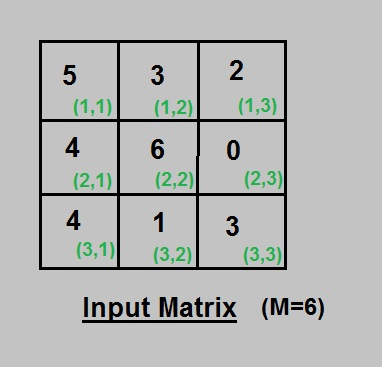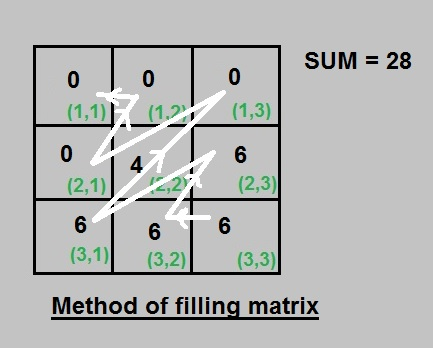# Maximize matrix as per given condition

You are given a N*N matrix, where each element of matrix lies in the range 0 to M. You can apply the below operation on matrix any number of times:

• Choose any two consecutive elements
• Increment one of them by 1 and decrease other by 1

Note: The elements should remain within the range 0 to M after applying above operations.
The task is to find the maximum value of the expression shown below that can be obtained after performing the above operation on matrix if required:

```res += (i+j)*A[i][j]

for 0 <= i, j <= N
```

Examples:

```Input : A[][] = {1, 2,
5, 1}
M = 5
Output : RESULT = 27
Matrix : 0 0
4 5

Input : A[][] = {3, 4,
5, 4}
M = 6
Output : RESULT = 43
Matrix : 0 4
6 6
```

## Recommended: Please try your approach on {IDE} first, before moving on to the solution.

Algorithm :
Below is the step by step algorithm to do this:

1. First of all, calculate the sum of all elements of given matrix as SUM.
2. Start from last element that i.e. A(n, n) and move backward towards A(0,0) anti-diagonally as A(n, n), A(n, n-1), A(n-1, n), A(n, n-2), A(n-1, n-1), A(n-2, n)…..
3. Fill up each cell of matrix with M and update SUM = SUM- M for each element till SUM < M. Now, Fill the SUM value at next place in order if it is greater than zero and all other remaining place as zero.
4. Finally you can calculate RESULT as per above mentioned formula.

Example :
Input Matrix:Solution Matrix after applying above algorithm :Below is the implementation of above idea :

## C++

 `// CPP to maximize matrix result ` `#include ` `using` `namespace` `std; ` `#define n 4 ` ` `  `// utility function for maximize matrix result ` `int` `maxMatrix(``int` `A[][n], ``int` `M) ` `{ ` `    ``int` `sum = 0, res = 0; ` `    ``for` `( ``int` `i=0; i0; j--) ` `    ``{ ` `        ``for` `(``int` `i=0; i M) ` `            ``{ ` `                ``A[n-1-i][j+i] = M; ` `                ``sum -= M; ` `            ``} ` `            ``else` `            ``{ ` `                ``A[n-1-i][j+i] = sum; ` `                ``sum -= sum; ` `            ``} ` `        ``} ` `    ``}   ` ` `  `    ``// diagonals above longest diagonal ` `    ``for` `(``int` `i=n-1; i>=0; i--) ` `    ``{ ` `        ``for` `(``int` `j=0; j<=i; j++) ` `         ``{ ` `            ``if` `(sum > M) ` `            ``{ ` `                ``A[i-j][j] = M; ` `                ``sum -= M; ` `            ``} ` `            ``else` `            ``{ ` `                ``A[i-j][j] = sum; ` `                ``sum -= sum; ` `            ``} ` `        ``} ` `    ``} ` ` `  `    ``// calculating result ` `    ``for` `(``int` `i=0; i

## Java

 `// Java to maximize matrix result  ` ` `  `class` `GFG { ` ` `  `    ``static` `final` `int` `n = ``4``; ` ` `  `// utility function for maximize matrix result  ` `    ``static` `int` `maxMatrix(``int` `A[][], ``int` `M) { ` `        ``int` `sum = ``0``, res = ``0``; ` `        ``for` `(``int` `i = ``0``; i < n; i++) { ` `            ``for` `(``int` `j = ``0``; j < n; j++) { ` `                ``sum += A[i][j]; ` `            ``} ` `        ``} ` ` `  `        ``// diagonals below longest diagonal  ` `        ``// starting from last element of matrix  ` `        ``for` `(``int` `j = n - ``1``; j > ``0``; j--) { ` `            ``for` `(``int` `i = ``0``; i < n - j; i++) { ` `                ``if` `(sum > M) { ` `                    ``A[n - ``1` `- i][j + i] = M; ` `                    ``sum -= M; ` `                ``} ``else` `{ ` `                    ``A[n - ``1` `- i][j + i] = sum; ` `                    ``sum -= sum; ` `                ``} ` `            ``} ` `        ``} ` ` `  `        ``// diagonals above longest diagonal  ` `        ``for` `(``int` `i = n - ``1``; i >= ``0``; i--) { ` `            ``for` `(``int` `j = ``0``; j <= i; j++) { ` `                ``if` `(sum > M) { ` `                    ``A[i - j][j] = M; ` `                    ``sum -= M; ` `                ``} ``else` `{ ` `                    ``A[i - j][j] = sum; ` `                    ``sum -= sum; ` `                ``} ` `            ``} ` `        ``} ` ` `  `        ``// calculating result  ` `        ``for` `(``int` `i = ``0``; i < n; i++) { ` `            ``for` `(``int` `j = ``0``; j < n; j++) { ` `                ``res += (i + j + ``2``) * A[i][j]; ` `            ``} ` `        ``} ` `        ``return` `res; ` `    ``} ` ` `  `// driver program  ` `    ``static` `public` `void` `main(String[] args) { ` `        ``int` `A[][] = {{``1``, ``2``, ``3``, ``4``}, ` `        ``{``5``, ``6``, ``7``, ``8``}, ` `        ``{``9``, ``1``, ``1``, ``2``}, ` `        ``{``3``, ``4``, ``5``, ``6``}}; ` `        ``int` `m = ``9``; ` `        ``System.out.println(maxMatrix(A, m)); ` `    ``} ` `} ` ` `  `// This code is contributed by Rajput-Ji `

## Python3

 `# Python to maximize matrix result ` `n ``=` `4` ` `  `# utility function for maximize ` `# matrix result ` `def` `maxMatrix(A, M): ` `    ``sum``, res ``=` `0``, ``0` `    ``for` `i ``in` `range``(n): ` `        ``for` `j ``in` `range``(n): ` `            ``sum` `+``=` `A[i][j] ` ` `  `    ``# diagonals below longest diagonal ` `    ``# starting from last element of matrix ` `    ``for` `j ``in` `range``(n ``-` `1``, ``0``, ``-``1``): ` `        ``for` `i ``in` `range``(n ``-` `j): ` `            ``if` `(``sum` `> M): ` `                ``A[n ``-` `1` `-` `i][j ``+` `i] ``=` `M ` `                ``sum` `-``=` `M ` `            ``else``: ` `                ``A[n ``-` `1` `-` `i][j ``+` `i] ``=` `sum` `                ``sum` `-``=` `sum` `                 `  `    ``# diagonals above longest diagonal ` `    ``for` `i ``in` `range``(n ``-` `1``, ``-``1``, ``-``1``): ` `        ``for` `j ``in` `range``(i ``+` `1``): ` `            ``if` `(``sum` `> M): ` `                ``A[i ``-` `j][j] ``=` `M ` `                ``sum` `-``=` `M ` `            ``else``: ` `                ``A[i ``-` `j][j] ``=` `sum` `                ``sum` `-``=` `sum` `                 `  `    ``# calculating result ` `    ``for` `i ``in` `range``(n): ` `        ``for` `j ``in` `range``(n): ` `            ``res ``+``=` `(i ``+` `j ``+` `2``) ``*` `A[i][j] ` `    ``return` `res ` ` `  `# Driver Code ` `if` `__name__ ``=``=` `'__main__'``: ` `    ``A ``=` `[[``1``, ``2``, ``3``, ``4``], ` `         ``[``5``, ``6``, ``7``, ``8``], ` `         ``[``9``, ``1``, ``1``, ``2``], ` `         ``[``3``, ``4``, ``5``, ``6``]] ` `    ``m ``=` `9` `    ``print``(maxMatrix(A, m)) ` ` `  `# This code is contributed by 29AjayKumar `

## C#

 `     `  `// C# to maximize matrix result  ` `using` `System; ` `public` `class` `GFG { ` `  `  `    ``static` `readonly` `int` `n = 4; ` `  `  `// utility function for maximize matrix result  ` `    ``static` `int` `maxMatrix(``int` `[,]A, ``int` `M) { ` `        ``int` `sum = 0, res = 0; ` `        ``for` `(``int` `i = 0; i < n; i++) { ` `            ``for` `(``int` `j = 0; j < n; j++) { ` `                ``sum += A[i,j]; ` `            ``} ` `        ``} ` `  `  `        ``// diagonals below longest diagonal  ` `        ``// starting from last element of matrix  ` `        ``for` `(``int` `j = n - 1; j > 0; j--) { ` `            ``for` `(``int` `i = 0; i < n - j; i++) { ` `                ``if` `(sum > M) { ` `                    ``A[n - 1 - i,j + i] = M; ` `                    ``sum -= M; ` `                ``} ``else` `{ ` `                    ``A[n - 1 - i,j + i] = sum; ` `                    ``sum -= sum; ` `                ``} ` `            ``} ` `        ``} ` `  `  `        ``// diagonals above longest diagonal  ` `        ``for` `(``int` `i = n - 1; i >= 0; i--) { ` `            ``for` `(``int` `j = 0; j <= i; j++) { ` `                ``if` `(sum > M) { ` `                    ``A[i - j,j] = M; ` `                    ``sum -= M; ` `                ``} ``else` `{ ` `                    ``A[i - j,j] = sum; ` `                    ``sum -= sum; ` `                ``} ` `            ``} ` `        ``} ` `  `  `        ``// calculating result  ` `        ``for` `(``int` `i = 0; i < n; i++) { ` `            ``for` `(``int` `j = 0; j < n; j++) { ` `                ``res += (i + j + 2) * A[i,j]; ` `            ``} ` `        ``} ` `        ``return` `res; ` `    ``} ` `  `  `// driver program  ` `    ``static` `public` `void` `Main() { ` `        ``int` `[,]A= {{1, 2, 3, 4}, ` `        ``{5, 6, 7, 8}, ` `        ``{9, 1, 1, 2}, ` `        ``{3, 4, 5, 6}}; ` `        ``int` `m = 9; ` `        ``Console.Write(maxMatrix(A, m)); ` `    ``} ` `} ` `  `  `// This code is contributed by Rajput-Ji `

## PHP

 ` 0; ``\$j``--) ` `    ``{ ` `        ``for` `(``\$i` `= 0; ``\$i` `< ``\$n` `- ``\$j``; ``\$i``++) ` `        ``{ ` `            ``if` `(``\$sum` `> ``\$M``) ` `            ``{ ` `                ``\$A``[``\$n` `- 1 - ``\$i``][``\$j` `+ ``\$i``] = ``\$M``; ` `                ``\$sum` `-= ``\$M``; ` `            ``} ` `            ``else` `            ``{ ` `                ``\$A``[``\$n` `- 1 - ``\$i``][``\$j` `+ i] = ``\$sum``; ` `                ``\$sum` `-= ``\$sum``; ` `            ``} ` `        ``} ` `    ``}  ` ` `  `    ``// diagonals above longest diagonal ` `    ``for` `(``\$i` `= ``\$n` `- 1; ``\$i` `>= 0; ``\$i``--) ` `    ``{ ` `        ``for` `(``\$j` `= 0; ``\$j` `<= ``\$i``; ``\$j``++) ` `        ``{ ` `            ``if` `(``\$sum` `> ``\$M``) ` `            ``{ ` `                ``\$A``[``\$i` `- ``\$j``][``\$j``] = ``\$M``; ` `                ``\$sum` `-= ``\$M``; ` `            ``} ` `            ``else` `            ``{ ` `                ``\$A``[``\$i` `- ``\$j``][``\$j``] = ``\$sum``; ` `                ``\$sum` `-= ``\$sum``; ` `            ``} ` `        ``} ` `    ``} ` ` `  `    ``// calculating result ` `    ``for` `(``\$i` `= 0; ``\$i` `< ``\$n``; ``\$i``++) ` `    ``{ ` `        ``for` `(``\$j` `= 0; ``\$j` `< ``\$n``; ``\$j``++) ` `            ``\$res` `+= (``\$i` `+ ``\$j` `+ 2) *  ` `                     ``\$A``[``\$i``][``\$j``]; ` `    ``} ` `    ``return` `\$res``; ` `} ` ` `  `    ``// Driver Code  ` `    ``\$A` `= ``array``(``array``(1, 2, 3, 4), ` `               ``array``(5, 6, 7, 8), ` `               ``array``(9, 1, 1, 2), ` `               ``array``(3, 4, 5, 6)); ` `    ``\$m` `= 9; ` `    ``echo` `maxMatrix(``\$A``, ``\$m``); ` `     `  `// This code is contributed by anuj_67. ` `?> `

Output:

```425
```

Attention reader! Don’t stop learning now. Get hold of all the important DSA concepts with the DSA Self Paced Course at a student-friendly price and become industry ready.

My Personal Notes arrow_drop_up

Improved By : vt_m, Rajput-Ji, 29AjayKumar

Article Tags :
Practice Tags :

Be the First to upvote.

Please write to us at contribute@geeksforgeeks.org to report any issue with the above content.